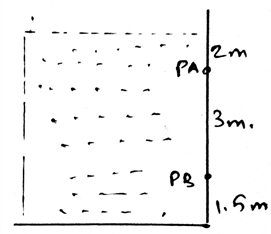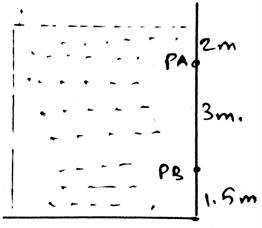Courses

# Test: Fluid Pressure

## 15 Questions MCQ Test Engineering Mechanics - Notes, Videos, MCQs & PPTs | Test: Fluid Pressure

Description
This mock test of Test: Fluid Pressure for Mechanical Engineering helps you for every Mechanical Engineering entrance exam. This contains 15 Multiple Choice Questions for Mechanical Engineering Test: Fluid Pressure (mcq) to study with solutions a complete question bank. The solved questions answers in this Test: Fluid Pressure quiz give you a good mix of easy questions and tough questions. Mechanical Engineering students definitely take this Test: Fluid Pressure exercise for a better result in the exam. You can find other Test: Fluid Pressure extra questions, long questions & short questions for Mechanical Engineering on EduRev as well by searching above.
QUESTION: 1

### The Pascal law states that liquid at rest applies pressure at a point is ________ in all directions.

Solution:

The Pascal law states that the liquid at rest applies pressure at a point is same in all directions. This means that the pressure is there in spite of the direction. And it is present in the same direction and is having same magnitude.

QUESTION: 2

### Determine the location of the hydrostatic force acting on the submerged rectangular plate PaPb. Plate has width of 1.5m. Density of water 1000kg/m3.Solution:

The Pascal law states that the liquid at rest applies pressure at a point is same in all directions. This means that the pressure is there in spite of the direction. And it is present in the same direction and is having same magnitude.

QUESTION: 3

### The magnitude of the pressure of the fluid is not dependent on the _________

Solution:

The Pascal law states that the liquid at rest applies pressure at a point is same in all directions. This means that the pressure is there in spite of the direction. And it is present in the same direction and is having same magnitude.

QUESTION: 4

The Pascal’s law is applicable to the liquid which is __________________

Solution:

The liquid must be incompressible. The Pascal law states that the liquid at rest applies pressure at a point is same in all directions. This means that the pressure is there in spite of the direction.

QUESTION: 5

Gases are ________________ fluids.

Solution:

The liquid must be incompressible. The Pascal law states that the liquid at rest applies pressure at a point is same in all directions. This means that the pressure is there in spite of the direction. But the gases are compressive that’s why it is not used in the gas form.

QUESTION: 6

Pressure acts _____________ to the surface of the body.

Solution:

The pressure is always acted normal to the surface of the body. Thus the pressure plays a role as a vector in this case. So mainly the pressure must be considered as normal to the surface of the body.

QUESTION: 7

For the determination of the fluid pressure the body to be experimented is ___________ in the liquid.

Solution:

The body used in the determination of the fluid pressure equations is immersed in the liquid. It is completely immersed in the liquid. So as to make the calculations of the pressure beneath the liquid.

QUESTION: 8

What is a collinear system of forces for the system of the fluid liquids?

Solution:

The force system having all the forces emerging from a point is called the collinear system of force. This is a type of system of the force, which is easy in the simplification. This is because as the forces are the vector quantity, the vector math is applied and the simplification is done.

QUESTION: 9

The system of the collinear and the parallel force for the calculations of the equations in the determination of the fluid pressure are simplified as ________________

Solution:

The simplification is easy. The simplified force system gives us a net force and the parallel force system gives us a simplified force, and then we add it vectorially. Thus the vector addition at the last can be easily done and thus both can be simplified.

QUESTION: 10

What is not the condition for the equilibrium in the determination of the equations of the fluid pressures?

Solution:

For the equilibrium in the three dimensional system of axis we have all the conditions true as, ∑Fx=0, ∑Fy=0 and ∑Fz=0. Also we have the summation of the forces equal to zero. Which is not a non-zero value.

QUESTION: 11

If solving the question in 3D calculations is difficult, then use the 2D system to determine the equations of the fluid pressure.

Solution:

The answer is obviously yes. If we are having any difficulty in making the vector components, then we can go in 2D. As if the particle is in equilibrium, the net force will be zero. No matter where you see first. Net force is zero.

QUESTION: 12

When the body is in equilibrium beneath the liquid then which of the following is true?

Solution:

Yes, we equate all the components of the three axis equal to zero. That is the resultant of the forces along the three axis are being equated to zero. This brings in that there is no net force along any direction. Hence equilibrium.

QUESTION: 13

State true or false. We first make equilibrium equations for the fluid pressure and then the free body diagram and then solve the question?

Solution:

We first make the free body diagram and then we make the equilibrium equations to satisfy the given conditions. This helps us to solve the question easily. As this reduces the part of imagination and increases accuracy too.

QUESTION: 14

∑Fx=0, ∑Fy=0 and ∑Fz=0 are vector equations for the determination of the equilibrium equations for the fluid pressure in the body immersed in the liquid.

Solution:

The answer is false as the equations asked are scalars. As we make the net sum of the forces along the axis equal to zero. Of course this equation comes from the solving the vector forms, but still the result is a scalar, hence the equations are scalar.

QUESTION: 15

Determine the resultant hydrostatic force acting on the submerged rectangular plate PaPb. Plate has width of 1.5m. Density of water 1000kg/m3.Solution:

The Pascal law states that the liquid at rest applies pressure at a point is same in all directions. This means that the pressure is there in spite of the direction. And it is present in the same direction and is having same magnitude.